# How to Subtract Decimal Numbers

How to Subtract Decimal Numbers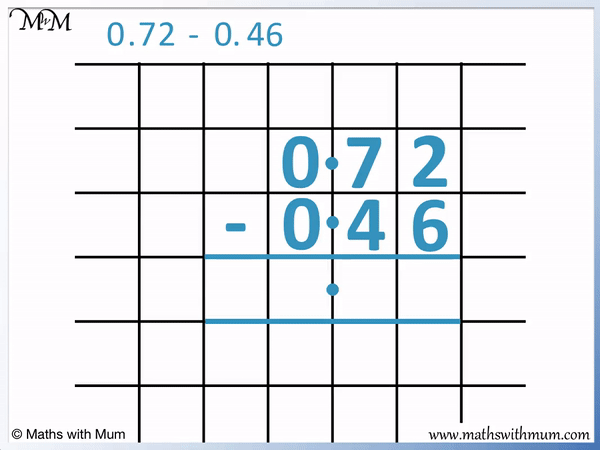• First line up the decimal points and the digits in each place value column.
• Write the number being subtracted below the other number.
• Subtract the digits in each column individually, starting from the right and moving left.
• Here, 6 is bigger than 2, so we need to borrow a ten from the 7 to do the subtraction.
• We decrease the digit we are borrowing from by 1 and add ten to the digit to its right.
• 7 – 1 = 6 and then 2 + 10 = 12.
• Now we can subtract 6 from 12. 12 – 6 = 6 and so we write 6 below.
• We subtract the digits in the column to the left. 6 – 4 = 2, so we write a 2 below.
• We write a decimal point in our answer in line with the decimal points above.
• In the ones column, 0 – 0 = 0 and so, we write a 0 below.
• 0.72 = 0.46 = 0.26.
Subtract each digit individually from right to left.

To borrow, subtract 1 from the digit to the left and add 10 to your digit.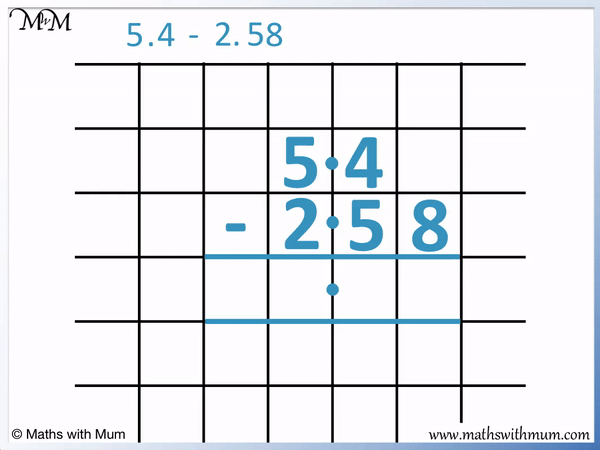• We line up the digits with 2.58 below 5.4.
• we put a 0 digit in after the 5.4 to make the decimals have the same length.
• 8 is larger than 0, so we need to borrow from the next column along.
• We subtract 1 from the 4 to make 3 and add 10 the 0 to make 10.
• Now we can subtract 8 from 10 to get 2, which we write below.
• 5 is larger than 3, so we need to borrow from the next column along.
• We subtract 1 from the 5 to make 4 and add 10 to the 3 to make 13.
• Now we can subtract 5 from 13 to get 8, which we write below.
• In the ones column we subtract 2 from 4 to get 2, which we write below.
• 5.4 – 2.58 = 2.82#### Column Subtraction Question Workbook

Download our printable workbooks for a wide range of practice of column subtraction, working up from basic questions through to subtracting decimals and money problems.

# Subtracting Decimals

## How to Subtract Decimals

To subtract decimals, follow these steps:

1. Line up the digits of the number being subtracted below the other number.
2. Put in zero digits to make the numbers the same length.
3. Subtract the digits in each place value column individually, working from right to left.
4. If the upper digit is smaller than the digit below it, regroup by subtracting 10 from the digit to its left and adding 10 to this digit.
5. Write the answer to each digit subtraction below to form the final answer.

Follow these steps if you need to subtract decimal numbers without a calculator.

Here is an example of subtracting decimals without regrouping.

We have 8.5 – 2.1.

The two decimal numbers have the same length, with one digit after the decimal point.

Write the decimal number being subtracted below the other number. We are subtracting 2.1 from 8.5 and so, we write 2.1 below 8.5.

Start by writing the numbers in line with each other by lining up the decimal places. The ones column digits are written in line with each other and the tenths column digits are written in line with each other.

Before doing any subtraction calculations, write the decimal point of the answer in directly below the other decimal points in the question.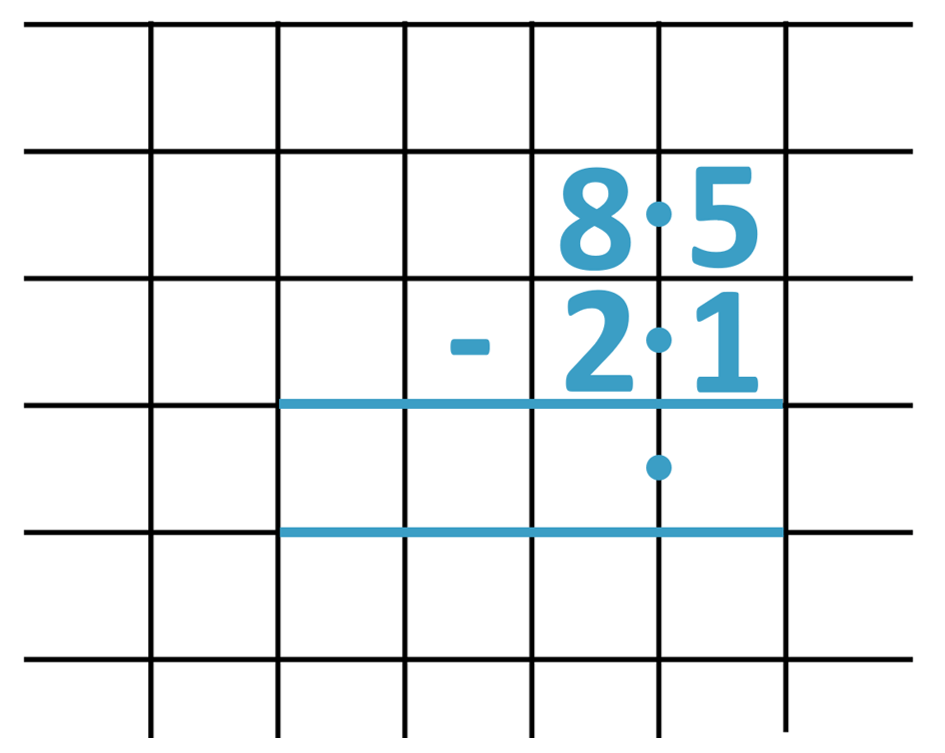The next step is to subtract the digits in each place value column, starting with the digits on the right. Subtract the digit below from the digit above.5 – 1 = 4 and so, we write the digit 4 in the answer space below.

We now subtract the digits in the ones column.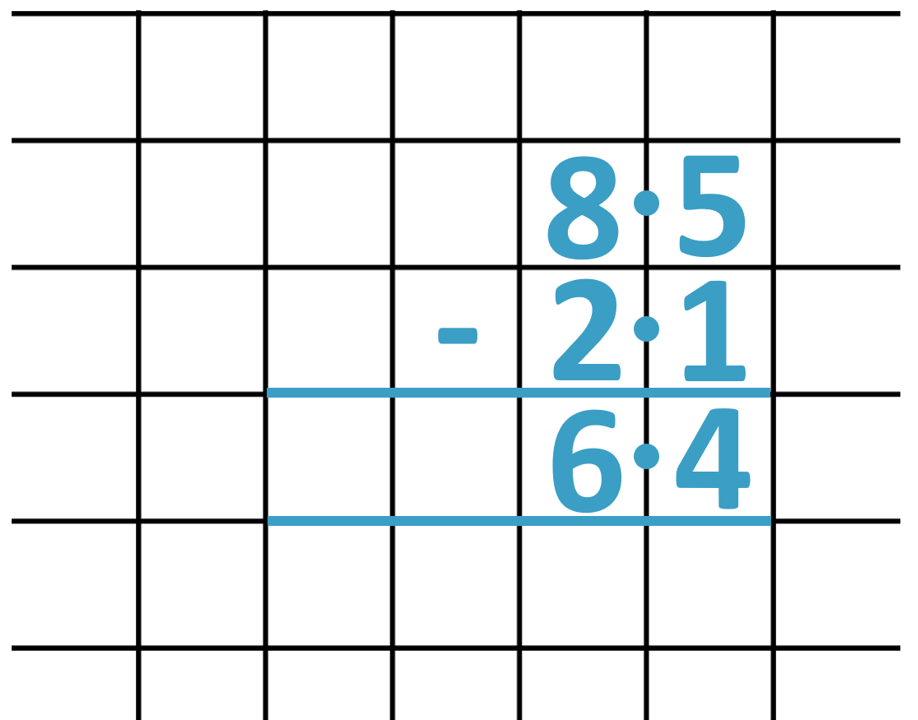8 – 2 = 6 and so, we write 6 in the space below.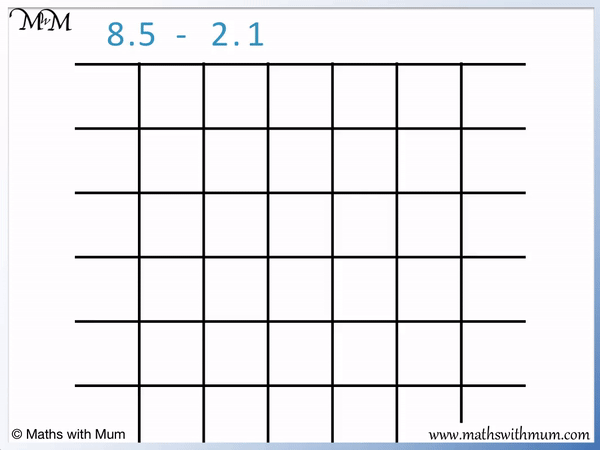When teaching subtracting without regrouping, it is important to ensure that the digits are lined up carefully. Use square grid paper and write each digit in each grid. Use a ruler to draw two lines below the question to write the answer in. To line up decimals correctly, write in the decimal point first, directly below the other decimal points. Then write each place value column in line one by one.

This method is called column subtraction or vertical subtraction and it is the best written method to use to subtract numbers without a calculator.

## How to Subtract Decimals with Regrouping

Regrouping is used when the digit being subtracted is larger than the digit above. To regroup, add ten to the digit on top and then subtract one from the digit that is in the place value column immediately to the left of this digit.

With subtraction, the process of regrouping is also sometimes called exchanging or borrowing. Exchanging, borrowing and regrouping are just different names for the same process.

Regrouping will be needed whenever any of the digits of a particular number are larger than the corresponding digits in the same place value columns of the number it is being subtracted from.

For example, here is 0.72 – 0.46.

In the hundredths column, the 6 is larger than the 2 it is being subtracted from.We cannot subtract 6 from 2 using this method because 6 is larger than 2. We need to regroup by borrowing ten from the tenths column.

To borrow in subtraction, add 10 to a digit and subtract 10 from the digit immediately to its left.

We add 10 to the 2 to make 12 and subtract 1 from the 7 to make 6.This borrowing works because 1 tenth is worth the same as 10 hundredths. By taking one tenth from the 7, we can add 10 to the number in the hundredths column.

After borrowing, the subtraction process can be completed as normal. 12 – 6 = 6.

We now subtract the digits in the tenths column. We use the new regrouped value of 6 now.6 – 4 = 2 and so, we write 2 in the answer space below.

0.72 – 0.46 = 0.26. Here is the full process of regrouping by borrowing a tenth shown below.## How to Subtract Decimals with Different Decimal Places

If the numbers contain a different number of digits after the decimal point, write zero digits at the end of the shorter decimal until there are the same number of decimal places in both numbers. Then subtract the digits in each place value column from right to left.

For example, 7.39 – 6.4 is written as 7.39 – 6.40 so that both decimal numbers have two decimal places.

7.39 has 2 decimal places, whereas 6.4 only has 1 decimal place. If the decimals have varying lengths, then zeros are written at the end of the shorter decimal to make it the same length.Now we subtract the digits in the hundredths column. 9 – 0 = 9, so we write a 9 in the hundredths column of the answer.

In the tenths column, 4 is larger than 3 so we borrow from the ones column. 7 – 1 = 6 and then 3 + 10 = 13.

We can now subtract 4 from 13. 13 – 4 = 9 and so, we write the answer of 9 in the tenths column.

In the ones column, 6 – 6 = 0.

Our answer is 7.39 – 6.4 = 0.99.

We can see that it is necessary to write zeros in when subtracting decimals of varying lengths in order to ensure that numbers can be regrouped correctly.

Here is an example of 5.4 – 2.58. 5.4 has 1 decimal place, whereas 2.58 has 2 decimal places.

To subtract decimals of different lengths, write zero digits on to the end of the shorter decimal until they are the same length.

We write 5.4 – 2.58 as 5.40 – 2.58.We subtract 1 tenth from the 4 tenths to make 3 tenths.

We add 10 to the 0 hundredths to make 10 hundredths.

Now we can subtract the digits in the hundredths column. 10 – 8 = 2 and so, we write 2 in below.

In the tenths column, 5 is larger than 3 so we need to borrow again.

We subtract 1 unit from 5 units to make 4 units.

We add 10 to 3 tenths to make 13 tenths.

We can now subtract the tenths column. 13 – 5 = 8, which we write below.

In the units column, 4 – 2 = 2.

5.4 – 2.58 = 2.82.

The method of subtraction with regrouping can be extended to all decimal numbers and is the best method to use if subtracting decimals without a calculator.Now try our lesson on Rounding Decimals to the Nearest Whole Number where we learn how to round decimals to their nearest whole number.